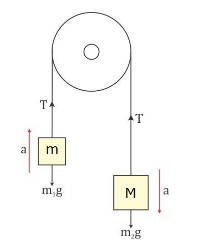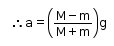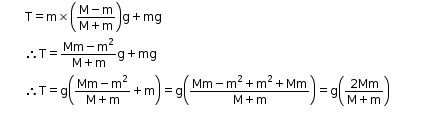# Two mass M and m are connected at two ends of an inextensible string

Two mass M and m are connected at two ends of an inextensible string. The string passes over a smooth frictionless pulley. Obtain the expression for the acceleration of the masses and the tension in the string. Given M>m.For mass m, the forces are given as
T - mg = ma … (1)
Similarly, for mass M, the forces are given as
Mg - T = Ma … (2)
From equation (1), we have
T = mg + ma … (3)
Substituting equation (3) in (2) we get
Mg - mg - ma = Ma
g(M - m) = a(M + m)Substituting the value of a in equation 1,